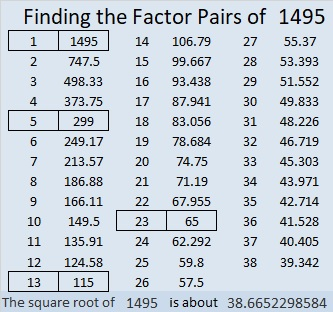# 1495 and Level 6

### Today’s Puzzle:

Hint: The only way 12 can be put in the first column of this puzzle is to let one of the 60’s use it. We don’t have to know which 60 that is, to know that a 5 will go above that 60 in the top row. Knowing that, where does 5 have to go in the first column? That’s the logic needed to get started on this puzzle:### Factors of 1495:

• 1495 is a composite number.
• Prime factorization: 1495 = 5 × 13 × 23.
• 1495 has no exponents greater than 1 in its prime factorization, so √1495 cannot be simplified.
• The exponents in the prime factorization are 1, 1, and 1. Adding one to each exponent and multiplying we get (1 + 1)(1 + 1)(1 + 1) = 2 × 2 × 2 = 8. Therefore 1495 has exactly 8 factors.
• The factors of 1495 are outlined with their factor pair partners in the graphic below.### Other Facts about the Number 1495:

1495 is the hypotenuse of FOUR Pythagorean triples:
368-1449-1495, which is 23 times (16-63-65)
575-1380-1495, which is (5-12-13) times 115,
759-1288-1495, which is 23 times (33-56-65), and
897-1196-1495, which is (3-4-5) times 299.